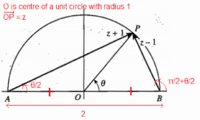# (z - 1)/(z + 1) on an Argand diagram

#### burgerandcheese

##### Junior Member
If z has modulus 1 and argument θ, where 0 < θ < π, find the modulus and argument of (z - 1)/(z + 1)I wanna know why the argument of (z + 1)/(z - 1) is "the angle you have to turn through to get from vector AP to vector BP, that is π/2 anticlockwise, or +π/2" What does that mean? Does it mean moving AP until it reaches BP? But isn't it always touching BP?

This came after learning the rules for multiplication and division in modulus-argument form

|st| = |s||t|, arg(st) = arg s + arg t + k(2π),
|s/t| = |s|/|t|, arg(s/t) = arg s - arg t + k(2π)

Last edited:

#### Subhotosh Khan

##### Super Moderator
Staff member
If z has modulus 1 and argument θ, where 0 < θ < π, find the modulus and argument of (z - 1)/(z + 1)

View attachment 12549

I wanna know why the argument of (z + 1)/(z - 1) is "the angle you have to turn through to get from vector AP to vector BP, that is π/2 anticlockwise, or +π/2" What does that mean? Does it mean moving AP until it reaches BP? But isn't it always touching BP?

This came after learning the rules for multiplication and division in modulus-argument form

|st| = |s||t|, arg(st) = arg s + arg t + k(2π),
|s/t| = |s|/|t|, arg(s/t) = arg s - arg t + k(2π)
That means "rotating" AP - centered at P - till it becomes co-linear (same-line) with BP.

#### Subhotosh Khan

##### Super Moderator
Staff member
•burgerandcheese